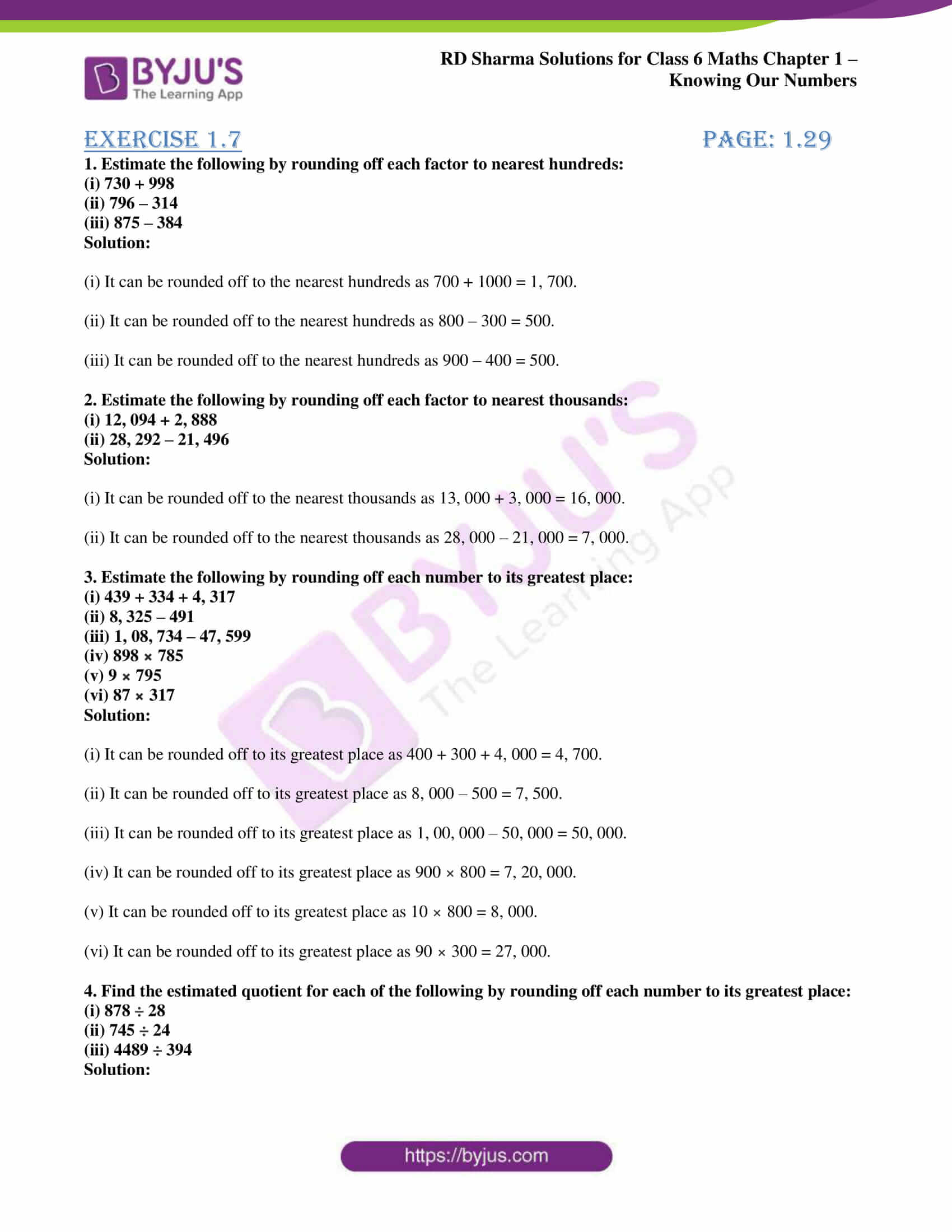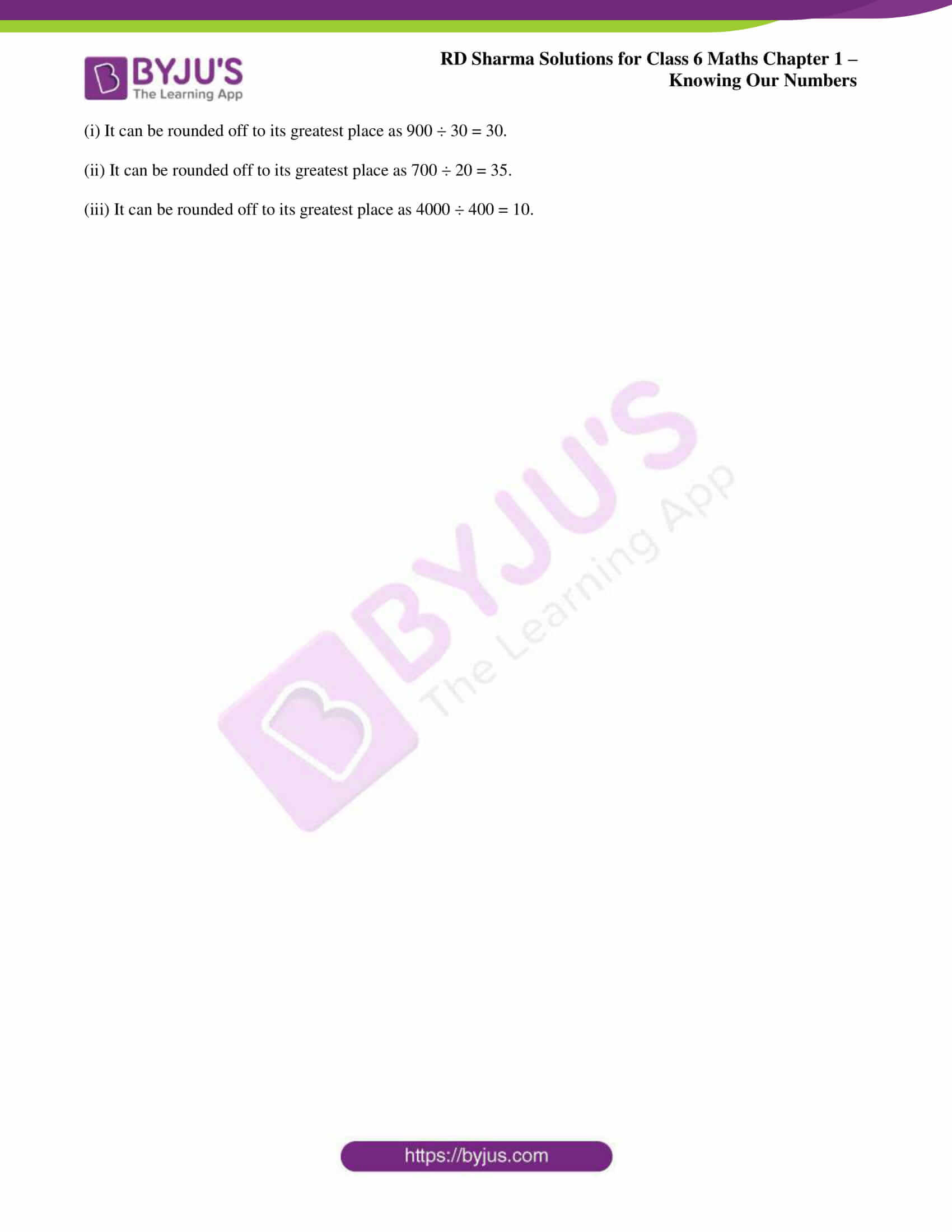# RD Sharma Solutions for Class 6 Maths Chapter 1: Knowing Our Numbers Exercise 1.7

## RD Sharma Solutions for Class 6 Maths Exercise 1.7 PDF

RD Sharma solutions are created by subject matter experts with explanations in a simple language to make them easy for students to understand. It helps students gain better knowledge about the method of solving problems and ace the exam. The students can use the PDF of solutions as a vital resource for revision purpose from the exam point of view. Solving the exercise wise problems using the solutions prepared by experts help in scoring good marks in the exams. RD Sharma Solutions for Class 6 Maths Chapter 1 Knowing Our Numbers Exercise 1.7 are provided here.

## RD Sharma Solutions for Class 6 Maths Chapter 1: Knowing Our Numbers Exercise 1.7 Download PDF## Access answers to Maths RD Sharma Solutions for Class 6 Chapter 1: Knowing Our Numbers Exercise 1.7

#### Exercise 1.7 page: 1.29

1. Estimate the following by rounding off each factor to nearest hundreds:

(i) 730 + 998

(ii) 796 – 314

(iii) 875 – 384

Solution:

(i) It can be rounded off to the nearest hundreds as 700 + 1000 = 1, 700.

(ii) It can be rounded off to the nearest hundreds as 800 – 300 = 500.

(iii) It can be rounded off to the nearest hundreds as 900 – 400 = 500.

2. Estimate the following by rounding off each factor to nearest thousands:

(i) 12, 094 + 2, 888

(ii) 28, 292 – 21, 496

Solution:

(i) It can be rounded off to the nearest thousands as 13, 000 + 3, 000 = 16, 000.

(ii) It can be rounded off to the nearest thousands as 28, 000 – 21, 000 = 7, 000.

3. Estimate the following by rounding off each number to its greatest place:

(i) 439 + 334 + 4, 317

(ii) 8, 325 – 491

(iii) 1, 08, 734 – 47, 599

(iv) 898 × 785

(v) 9 × 795

(vi) 87 × 317

Solution:

(i) It can be rounded off to its greatest place as 400 + 300 + 4, 000 = 4, 700.

(ii) It can be rounded off to its greatest place as 8, 000 – 500 = 7, 500.

(iii) It can be rounded off to its greatest place as 1, 00, 000 – 50, 000 = 50, 000.

(iv) It can be rounded off to its greatest place as 900 × 800 = 7, 20, 000.

(v) It can be rounded off to its greatest place as 10 × 800 = 8, 000.

(vi) It can be rounded off to its greatest place as 90 × 300 = 27, 000.

4. Find the estimated quotient for each of the following by rounding off each number to its greatest place:

(i) 878 ÷ 28

(ii) 745 ÷ 24

(iii) 4489 ÷ 394

Solution:

(i) It can be rounded off to its greatest place as 900 ÷ 30 = 30.

(ii) It can be rounded off to its greatest place as 700 ÷ 20 = 35.

(iii) It can be rounded off to its greatest place as 4000 ÷ 400 = 10.

### RD Sharma Solutions Class 6 Maths Chapter 1 – Knowing Our Numbers Exercise 1.7

RD Sharma Solutions Class 6 Maths Chapter 1 Knowing Our Numbers Exercise 1.7 helps estimation or rounding off the given numbers using sum, difference, product and quotient methods.

### Key features of RD Sharma Solutions for Class 6 Maths Chapter 1: Knowing Our Numbers Exercise 1.7

• The solutions are created with the aim of bridging the gap between the students and education.
• The brief explanation of solutions provide a clear idea about the topics and methods which are used in solving problems.
• The students can self evaluate their performance by solving problems using RD Sharma solutions.
• The solutions PDF created by faculty at BYJU’S help students to perform better in the exam.